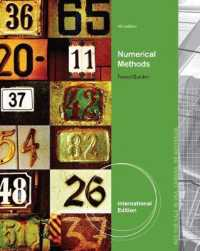Numerical Methods, International Edition -- Paperback / softback （4 ed）

• 在庫がございません。海外の書籍取次会社を通じて出版社等からお取り寄せいたします。
通常6～9週間ほどで発送の見込みですが、商品によってはさらに時間がかかることもございます。
重要ご説明事項
1. 納期遅延や、ご入手不能となる場合がございます。
2. 複数冊ご注文の場合、分割発送となる場合がございます。
3. 美品のご指定は承りかねます。
• 製本 Paperback:紙装版/ペーパーバック版
• 商品コード 9780495385691

Full Description

NUMERICAL METHODS, 4E, International Edition emphasizes the intelligent application of approximation techniques to the type of problems that commonly occur in engineering and the physical sciences. Readers learn why the numerical methods work, what kinds of errors to expect, and when an application might lead to difficulties. The authors also provide information about the availability of high-quality software for numerical approximation routines. The techniques are the same as those covered in the authors' top-selling Numerical Analysis text, but this text provides an overview for students who need to know the methods without having to perform the analysis. This concise approach still includes mathematical justifications, but only when they are necessary to understand the methods. The emphasis is placed on describing each technique from an implementation standpoint, and on convincing the reader that the method is reasonable both mathematically and computationally.

Contents

1. MATHEMATICAL PRELIMINARIES AND ERROR ANALYSIS. Introduction. Review of Calculus. Round-off Error and Computer Arithmetic. Errors in Scientific Computation. Computer Software. Introduction. The Bisection Method. The Secant Method. Newton's Method. Error Analysis and Accelerating Convergence. Muller's Method. Survey of Methods and Software. 3. INTERPOLATION AND POLYNOMIAL APPROXIMATION. Introduction. Lagrange Polynomials. Divided Differences. Hermite Interpolation. Spline Interpolation. Parametric Curves. Survey of Methods and Software. 4. NUMERICAL INTEGRATION AND DIFFERENTIATION. Introduction. Basic Quadrature Rules. Composite Quadrature Rules. Romberg Integration. Gaussian Quadrature. Adaptive Quadrature. Multiple Integrals. Improper Integrals. Numerical Differentiation. Survey of Methods and Software. Introduction. Taylor Methods. Runge-Kutta Methods. Predictor-Corrector Methods. Extrapolation Methods. Adaptive Techniques. Methods for Systems of Equations. Stiff Differentials Equations. Survey of Methods and Software. 6. DIRECT METHODS FOR SOLVING LINEAR SYSTEMS. Introduction. Gaussian Elimination. Pivoting Strategies. Linear Algebra and Matrix Inversion. Matrix Factorization. Techniques for Special Matrices. Survey of Methods and Software. 7. ITERATIVE METHODS FOR SOLVING LINEAR SYSTEMS. Introduction. Convergence of Vectors. Eigenvalues and Eigenvectors. Conjugate Gradient Methods. The Jacobi and Gauss-Seidel Methods. The SOR Method. Error Bounds and Iterative Refinement. Survey of Methods and Software. 8. APPROXIMATION THEORY. Introduction. Discrete Least Squares Approximation. Continuous Least Squares Approximation. Chebyshev Polynomials. Rational Function Approximation. Trigonometric Polynomial Approximation. Fast Fourier Transforms. Survey of Methods and Software. 9. APPROXIMATING EIGENVALUES. Introduction. Isolating Eigenvalues. The Power Method. Householder's Method. The QR Method. Survey of Methods and Software. Introduction. Newton's Methods for Systems. Quasi-Newton Methods. The Steepest Descent Method. Survey of Methods and Software. Homotopy and Continuation Methods.11. BOUNDARY-VALUE PROBLEMS FOR ORDINARY DIFFERENTIAL EQUATIONS. Introduction. The Linear Shooting Method. Linear Finite Difference Methods. The Nonlinear Shooting Method. Nonlinear Finite-Difference Methods. Variational Techniques. Survey of Methods and Software. 12. NUMERICAL METHODS FOR PARTIAL DIFFERENTIAL EQUATIONS. Introduction. Finite-Difference Methods for Elliptic Problems. Finite-Difference Methods for Parabolic Problems. Finite-Difference Methods for Hyperbolic Problems. Introduction to the Finite-Element Method. Survey of Methods and Software.